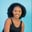Related Tags

dart
communitycreator

# How to use the fat arrow notation in Dart programmingMaria Elijah

Dart uses the fat arrow notation (=>) to define a single expression in a function. It is a neat method to write a single-line function expression.

### Syntax

ReturnType FuncName(Params) => Expression;


The code snippet above contains the following components:

• ReturnType: contains datatypes such as void, int, bool, and so on.

• FuncName: denotes the name of the function.

• Params: represents the set of parameters that the function requires.

• Expression: is the single statement to be executed.

When the function’s body is only one line long, you can use the fat arrow instead of the curly brackets and the return statement.

Note: Body {} statements do not exist in fat arrow notation. The body of the statement is replaced with the => symbol that points to a single statement.

### Code

The code sample below does not use the fat arrow notation:

// A function that calculates the area of a triangle

void areaOfaTriangle(int base, int height) {
var area = 0.5 * base * height;
print('Area of a triangle: $area'); } void main() { areaOfaTriangle( 7, 12); }  The areaOfaTriangle() function from the code snippet above can also be written using the fat arrow notation, as shown below: // A function that calculate area of a triangle // using Fat arrow notation void areaOfaTriangle(int base, int height) => print('Area of a triangle:${0.5 * base * height}');

void main() {
areaOfaTriangle( 7, 12);
}   

The code above produces the same output with fewer lines of code.

RELATED TAGS

dart
communitycreator

CONTRIBUTORMaria Elijah
RELATED COURSES

View all Courses

Keep Exploring

Learn in-demand tech skills in half the time